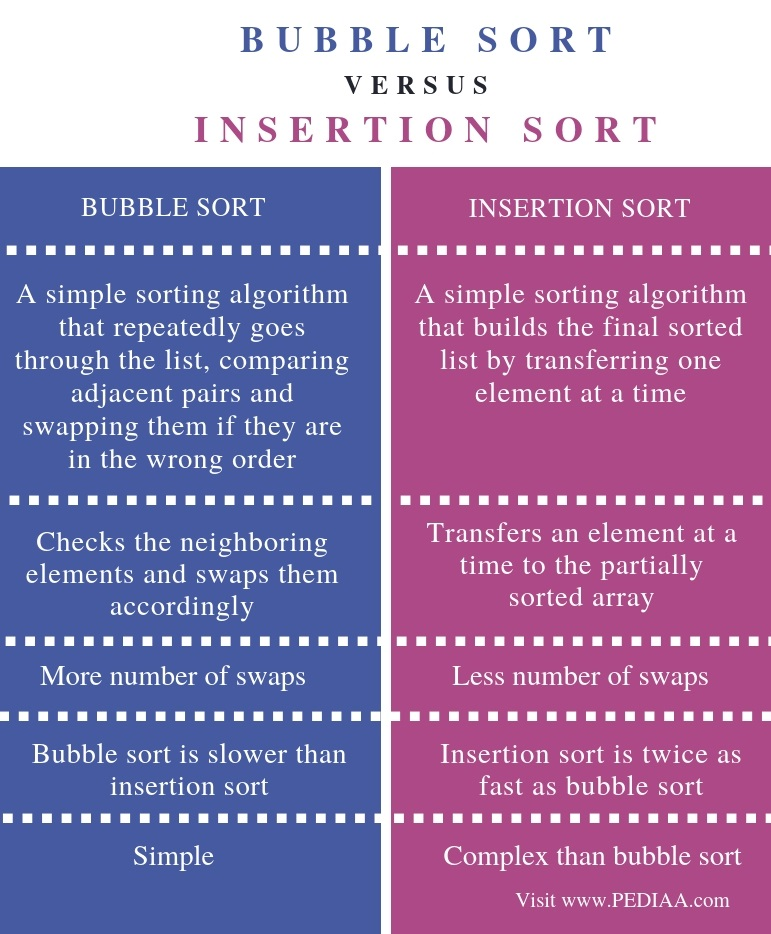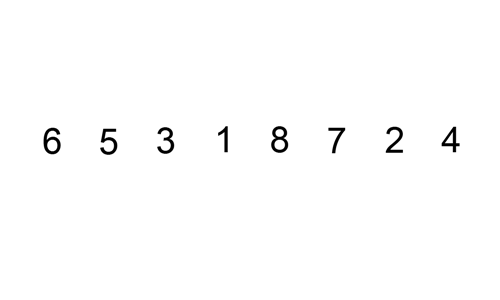# What is the Difference Between Bubble Sort and Insertion Sort

The main difference between bubble sort and insertion sort is that bubble sort performs sorting by checking the neighboring data elements and swapping them if they are in wrong order while insertion sort performs sorting by transferring one element to a partially sorted array at a time.

An algorithm is a sequence of steps to solve a problem. Sorting is a common operation to perform on a data set. There are various algorithms to sort a data set. Two of them are bubble sort and insertion sort. Moreover, these two algorithms are considered as simple sorting algorithms.

### Key Areas Covered

1. What is Bubble Sort
– Definition, Functionality
2. What is Insertion Sort
– Definition, Functionality
3. Difference Between Bubble Sort and Insertion Sort
– Comparison of Key Differences

### Key Terms

Algorithm, Bubble Sort, Insertion Sort## What is Bubble Sort

Bubble sort is the simplest sorting algorithm. The algorithm sorts the elements by comparing the adjacent pairs at a time.Consider the following example:

40  30  10  70   50  20  60

In bubble sorting, we compare neighboring elements.

First, we consider 40 and 30. 30 is less than 40. So, we can swap those two numbers.

30  40  10  70  50  20  60

Now, we can consider 40 and 10. 10 is less than 40. So, we can swap those two numbers.

30  10  40  70  50  20  60

Now, we can consider 40 and 70. As 70 is greater than 40, there is no need for swapping the numbers.

Next, we consider 70 and 50. 50 is less than 70. So, we can swap those two numbers.

30  10  40  50  70  20  60

Then, we can consider 70 and 20.  As 20 is less than 70, we can swap those two elements.

30  10  40  50  20  70  60

Now, we can consider 70 and 60. 60 is less than 70. Therefore, we have to swap those two numbers.

30  10  40  50  20  60  70

Now, you can see that the largest element in the data set is now at the end. In other words, at the end of the first pass, the largest element is already sorted. Therefore, next time, we do not have to consider 70 as it is already sorted. We only have to check the other six elements.

Moreover, we have to perform comparing two elements at a time. Consider 30 and 10. 10 is less than 30. So, we swap those two numbers.

10  30  40  50  20  60  70

Now, we consider 30 and 40. 40 is greater than 30. There is no need for swapping the numbers. Then, we can consider 40 and 50. As 50 is greater than 40, there is no need for swapping.

Now, consider 50 and 20. 20 is less than 50. So, we swap those two numbers.

10  30  40  20  50  60  70

Now, consider 50 and 60. There is no need for swapping. At the end of the second pass, the second largest element is sorted. In other words, 60 and 70 are now sorted. The process continues until sorting all the elements.

## What is Insertion Sort

Insertion sort algorithm sorts the dataset by transferring one element at a time to the partially sorted array.  Thus, this sorting algorithm has a low overhead.Consider the following example:

40  30  10  70   50  20  60

We consider 40 as the partially sorted array. When we consider 30, it is less than 40. So we swap them. Then, we consider 30 and 40 are in the partially sorted array.

30  40  10  70  50  20  60

Now, we consider 10. 10 is less than 30. So, we place the elements as below. 10, 30 and 40 are in the partially sorted array.

10  30  40  70  50  20  60

Now, we consider 70.  It is greater than 40, so there is no need for any movement. 10, 30, 40, 70 are in the partially sorted array.

Now, consider 50. It is less than 70 but greater than 40. We can place them in the correct position. 10,30, 40, 50,70 are now in the partially sorted array.

10  30  40  50  70  20  60

Now, consider 20. It is greater than 10 but less than 20. We can place it in the correct position. 10,20,30,40,50, 70 are in the partially sorted array.

10 20  30  40  50  70  60

Consider 60. It is less than 70 but greater than 50. We can place it in the correct position.

10  20  30  40  50  60  70

Now, we can see that all the elements are sorted. Here, the number of swaps in insertion sort is minimized but the number of comparisons is still high.

## Difference Between Bubble Sort and Insertion Sort

### Definition

Bubble sort is a simple sorting algorithm that repeatedly goes through a list, comparing adjacent pairs and swapping them if they are in the wrong order. Insertion sort, on the other hand, is a simple sorting algorithm that builds the final sorted list by transferring one element at a time. Thus, this is the main difference between bubble sort and insertion sort.

### Functionality

While bubble sort checks the neighboring elements and swaps them accordingly, insertion sort transfers an element at a time to the partially sorted array.

### Number of swaps

Also, the number of swaps is an important difference between bubble sort and insertion sort. Insertion sort has less number of swaps compared to bubble sort.

### Speed

Moreover, insertion sort is twice as fast as bubble sort.

### Complexity

Another difference between bubble sort and insertion sort is that insertion sort is more complex than bubble sort.

### Conclusion

Bubble sort and insertion sort is suitable for sorting a small dataset. Both have lower efficiency when compared to other advanced sorting algorithms such as quicksort and merge sort. The main difference between bubble sort and insertion sort is that bubble sort performs sorting by checking the neighboring data elements and swapping them if they are in wrong order while insertion sort performs sorting by transferring one element to the partially sorted array at a time.

##### References:

1.“Bubble Sort.” Wikipedia, Wikimedia Foundation, 15 Apr. 2019, Available here.
2.“Insertion Sort.” Wikipedia, Wikimedia Foundation, 3 Feb. 2019, Available here.
3.“What Is an Insertion Sort? – Definition from Techopedia.” Techopedia.com, Available here.

##### Image Courtesy:

1.1.”2816806″ (CC0) via Pixabay